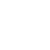Classes (extension) |

# KMeansRT : UGen : AbstractFunction : ObjectExtension

k-means classification in real time
Source: SOMTrain.sc

## Description

Performs online k-means classification, classifying each datapoint that comes in and updating the k-means centroids.

The method is described in Algorithm B.1 of Brian McFee's 2012 thesis (http://cseweb.ucsd.edu/~bmcfee/papers/bmcfee_dissertation.pdf ). McFee calls it "online Hartigan k-means".

## Class Methods

### KMeansRT.kr(bufnum, inputdata, k: 5, gate: 1, reset: 0, learn: 1)

#### Arguments:

 bufnum a Buffer with "k" frames and "d + 1" channels (where d is the number of dimensions of input data. Each frame of the Buffer will hold data for a centroid (the centroid location, plus the final channel holds the effective number of points associated with the centroid). inputdata An array representing the input point. Num channels must match the dimensionality of the points in the dataset. k k is the number of centroids. gate The unit is active while gate > 0. While <=0, no search is performed and output is held steady reset If reset is greater than 0, the "flexibility" of the centroids is reset back to initial values (as if no data had been received). learn Controls whether the unit is learning from its input. If you set this to zero it will not learn, but will still output decisions. This is useful for applying a previously-learned clusterer without modifying it. This argument cannot be modulated: each time you use KMeansRT it is either learning from scratch, or using a fixed pre-learnt buffer.

#### Returns:

the cluster index with which the input datapoint has been associated.

### KMeansRT.getCentroid(bufnum, classif, ndims)

A convenience method (just a wrapper round BufRd really) that lets you access the centroid location, given a cluster index.

#### Arguments:

 bufnum the same buffer as is passed to .kr classif the classification index, i.e. the output from .kr ndims the number of dimensions ("d" in the above description)

#### Returns:

the "d"-dimensional current location of the centroid corresponding to index "classif".

## Examples

This example clusters every frame of a sound sample, using the spectral centroid of each frame, and sonifies the result:

This example clusters onsets: